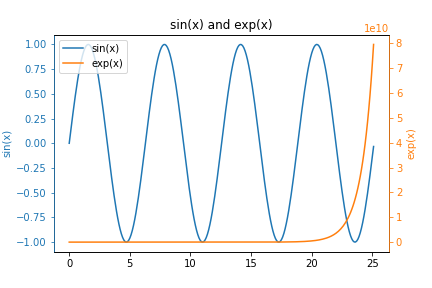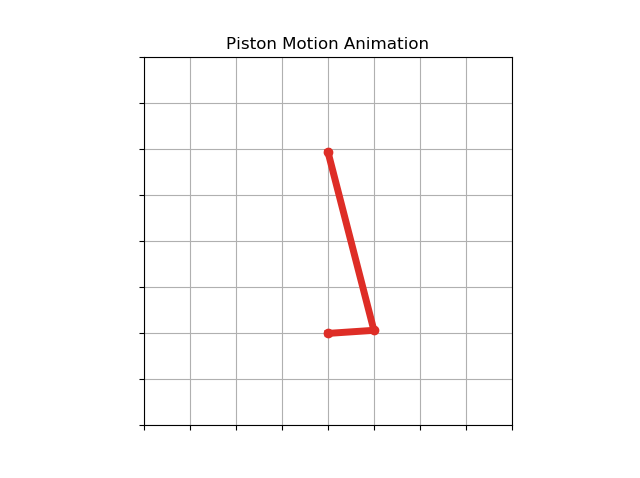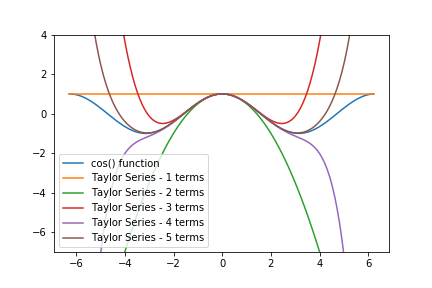## How to make y-y plots with MatplotlibIn this post, you will learn how to create y-y plots with Python and Matplotlib. y-y plots are a type of line plot where one line corresponds to one y-axis and another line on the same plot corresponds to a different y-axis. y-y plots typically have one vertical y-axis on …

## Matplotlib Animation to Demonstrate Piston MotionPiston motion is one of the classic types of motion in engineering dynamics that belong to a category of 4-bar motion. Piston motion is the type of motion that the piston in a cylinder of a car engine goes through as the engine's crankshaft rotates. In this post, we'll review …

## Plotting a Stress Strain Curve with Python and MatplotlibIn this post, we'll use data from a tensile test to build a stress strain curve with Python and Matplotlib.

A tensile test is a type of mechanical test performed by engineers used to determine the mechanical properties of a material. Engineering metal alloys such as steel and aluminum alloys …

## Plotting Bond Energy vs. Distance with Python and MatplotlibAtoms in solid materials like steel and aluminum are held together with chemical bonds. Atoms of solid materials are more stable when they are chemically bonded together, and it takes energy to separate atoms which are joined together with a chemical bond. The bonding energy associated with a chemical bond …

## Taylor Series in PythonIn this post, we will review how to create a Taylor Series with Python and for loops. Then we will refactor the Taylor Series into functions and compare the output of our Taylor Series functions to functions from Python's Standard Library.

A Taylor Series is an infinite series of mathematical …